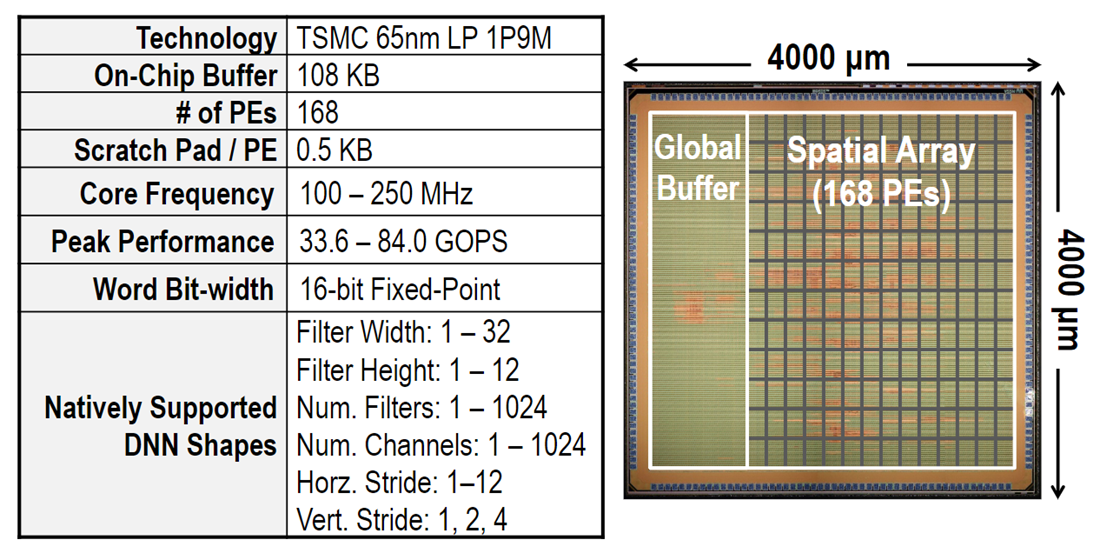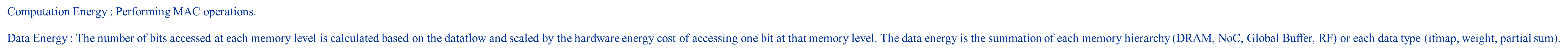# Energy-Estimator-for-Eyeriss-like-Architecture-

An energy estimator for eyeriss-like DNN hardware accelerator

This is an energy estimator for eyeriss-like architecture utilizing Row-Stationary dataflow which is a DNN hardware accelerator created by works from Vivienne Sze’s group in MIT. You can refer to their original works in github, Y. N. Wu, V. Sze, J. S. Emer, “An Architecture-Level Energy and Area Estimator for Processing-In-Memory Accelerator Designs,” IEEE International Symposium on Performance Analysis of Systems and Software (ISPASS), April 2020, http://eyeriss.mit.edu/, etc. Thanks to their contribution in DNN accelerator and energy efficient design.Eyeriss-like architecture utilizes row-stationary dataflow in order to fully explore data reuse including convolutional reuse, ifmap reuse and filter reuse. In general, the energy breakdown in each DNN layer can be separated in terms of computation and memory access (or data transfer).Computation Energy : Performing MAC operations. Data Energy : The number of bits accessed at each memory level is calculated based on the dataflow and scaled by the hardware energy cost of accessing one bit at that memory level. The data energy is the summation of each memory hierarchy (DRAM, NoC, Global Buffer, RF) or each data type (ifmap, weight, partial sum).1. Quantization

Bitwidth Energy scaling in computation : linear for single operand scaling. Quadratic for two operands scaling. Energy scaling in data access : Linear scaling for any data type in any memory hierarchy.
2. Pruning on filters (weights) Energy scaling in computation : Skip MAC operations according to pruning ratio. (Linear scaling) Energy scaling in data access : Linear scaling for weight access.Assumptions: Initial image input and weights in each layer should be first read from DRAM (external off-chip memory). Global Buffer is big enough to store any amount of datum and intermediate numbers. NoC has high-performance and high throughput with non-blocking broadcasting and inter-PE forwarding capability which supports multiple information transactions simultaneously. No data compression technique is considered in estimator design. Each PE is able to recognize information transferred among NoCs so that only those in need could receive data. Sparsity of weights and activations aren’t considered. Register File inside each PE only has the capacity to store one row of weights, one row of ifmap and one partial sum which means that we won’t take the capacity of RF into account. (A pity in this energy estimator) Ifmap and ofmap of each layer should be read from or written back into DRAM for external read operations. Once a data value is read from one memory level and then written into another memory level, the energy consumption of this transaction is always decided by the higher-cost level and only regarded as a single operation. Data transfer could happen directly between any 2 memory levels. This estimator is only applied to 2D systolic PE arrays. Partial sum and ofmap of one layer have the same bitwidth as activations. Maxpooling, Relu and LRN are not taken into account with respect to energy estimation. (little impact on total estimation) In order to make full use of data reuse (convolutional reuse and ifmap reuse), apart from row-stationary dataflow, scheduling algorithm will try to avoid reading ifmaps as much as possible. Once a channel of ifmap is kept inside the RF, the computation will be executed across the corresponding channel of entire filters in each layer.

Example analysis : Hardware Architecture : Eyeriss PE size : 12*14, 2D Dataflow : Row Stationary DNN Model : AlexNet (5 conv layers, 3 FC layers) Initial Input : single image from ImageNet Additional Attributes : Pruning and Quantization (You can revise your own pruning ratio and bitwidth of weight and activation in my source code) Everything is not hard-coded !

A pity ! (future works to do) 3D PE arrays. Memory size is considered in scheduling algorithm to accommodate more intermediate datum in low-cost level without writing back to high-cost level. Possible I/O data compression. (encoder, decoder) Possible sparsity optimization. (zero-gated MAC) Elaborate operation with specific arguments like random read, repeated write, constant read, etc. The impact of memory size, spatial distribution, location can be taken into account when we try to improve precision of our energy estimator. For example, the spatial distribution between two PEs can be characterized by Manhattan distance which can be used to scale the energy consumption of data forwarding in NoC.